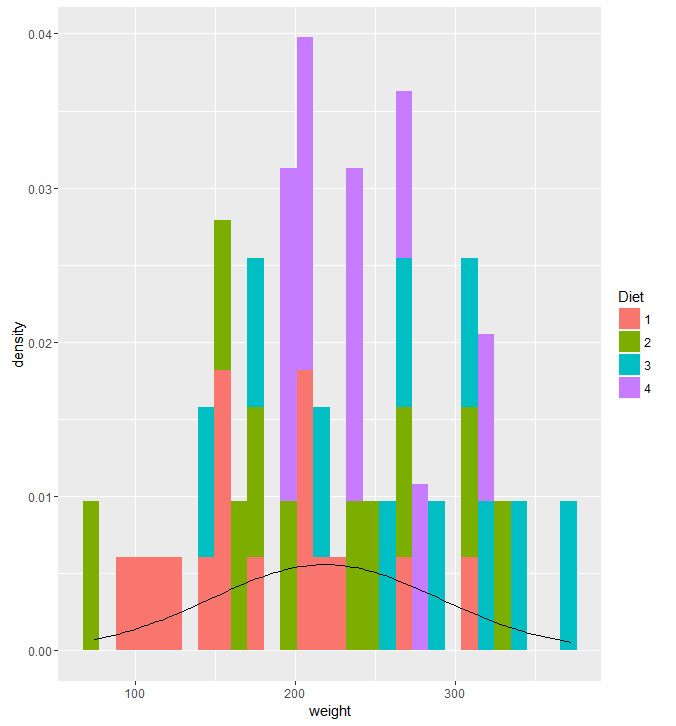Select PageHere is a scatter plot by @KenSteif on twitter that shows the distribution of the price of LEGO sets.  On top of the graph, Ken has super imposed a regression line to show the trend of the cost.  From the graph you can see that the data is ungrouped and that there are a few outliers in the top left and far right areas.  However, the scatterplot is generally homoscedastic.`ggplot(df, aes(x=weight, fill = Diet)) + geom_histogram(aes(y = ..density..)) + stat_function(fun=dnorm, args=list(mean=mean(df\$weight), sd=sd(df\$weight)))`

I tried to make a histogram out of the ChickWeight dataset by looking at the weights on the final day and coloring them by their diet.  However, adding the normal curve didn’t go well.  I wanted the graph to display the counts and have the normal curve scaled to an appropriate Y value, but I didn’t make any significant progress.  Most of the responses I saw on how to do this reset the Y axis to density, then plot the density curve.  It really bugs me that I didn’t have a quick or easy solution to this given my experience with R.  Ggplot2 is a system that I am aware I need to work on, and am actively trying to work on.